# Multiplication by 1 Digit Number

## NUMBERS AND OPERATIONSThe multiplication is that operation that consists of calculating the product (result) that is obtained by adding the same number (factor or multiplicand) so many times as the value of the other number (factor or multiplicator or multiplier).

Multiplication is used when we have to replace the calculation of certain repetitive additions (for example: 2 + 2 + 2 + 2 = 8) by a more efficient method (2 x 4 = 8).

In general, multiplication is considered the inverse operation of division.

#### Multiply natural numbers without carrying: 3 digits by 1 digit

We propose you some school tasks with the numbers up to 999 in the multiplicand together with their detailed explanation so that you understand step by step how to solve three-digit multiplications for a number without carry.

SPANISHENGLISHLook at the solved multiplication that we propose and you will learn how to calculate a multiplication of three digits in the multiplicand by one digit in the multiplier without regrouping: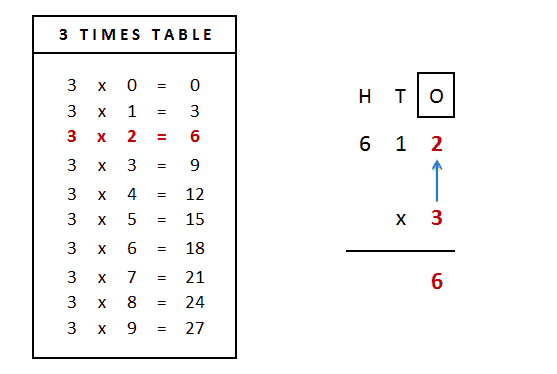Let's multiply 612 x 3

1st) We start by multiplying the multiplier (lower digit) by the number of the ones of the multiplicand (upper digit):

3 x 2 = 6 ones

2nd) Next, we will write the result of the multiplication (6) below the horizontal dividing line and aligning it with the figure of the ones.

3rd) We will multiply, on this occasion, the figure of the multiplier (table of 3) by the figure of the tens of the multiplicand:

3 x 1 = 3 tens

4th) Next, we write the result of the multiplication (3) below the figure of the tens in an aligned way and below the horizontal dividing line.5th) We continue multiplying the multiplier (lower figure) by the digit of the hundreds of the multiplicand (upper figure):

3 x 6 = 18 hundreds

6th) Finally, we write the result of the multiplication (18) below the figure of the hundreds and after the horizontal dividing line.

You have finally solved the multiplication! The result: 612 x 3 = 1,836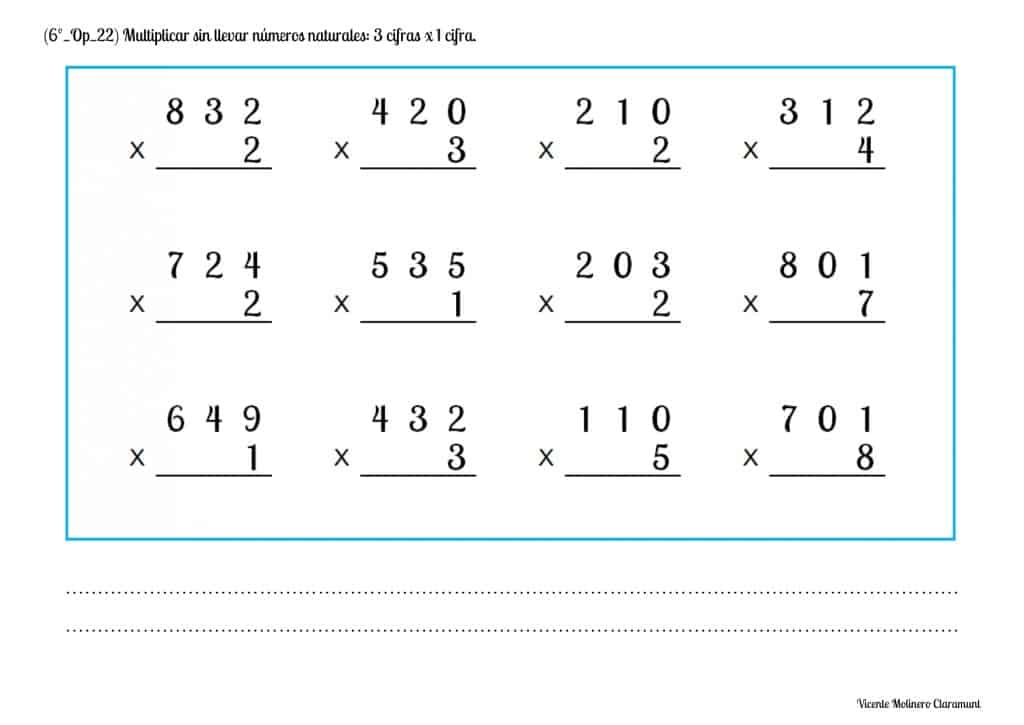#### Solve multiplication word problems without carrying with natural numbers: 3 digits x 1 digit

We provide you with a variety of multiplication word problems of three figures by one figure (without regrouping) so that you can continue to improve your calculation skills and solving word problem situations.

SPANISHSPANISHENGLISH#### Multiply natural numbers with carrying: 3 digits by 1 digit

We offer you some practical exercises prepared for downloading and printing in PDF supported by a pedagogical tutorial so that you quickly understand how to find the multiplications by carrying over of three digits (multiplicand) by one digit (multiplier).

SPANISHENGLISHWe show you a solved example that will help you learn step by step how to solve three-digit multiplication in the multiplicand by one digit in the multiplier and regrouping: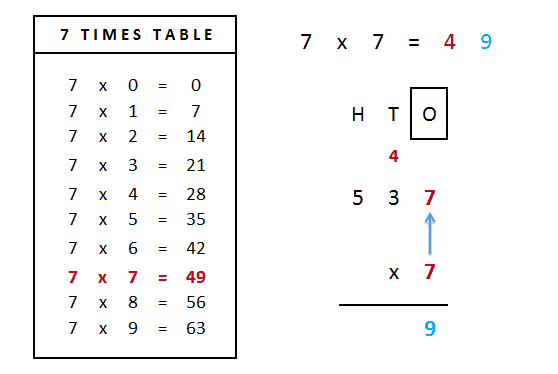Let's multiply 537 x 7

1st) We start by multiplying the multiplier (lower digit) by the number of the ones of the multiplicand (upper digit):

7 x 7 = 49 ones

2nd) The 49 represents 4 tens and 9 ones. We will align the digit 9 below the dividing line (since it corresponds to the ones) and we will save the 4 tens in the tens column to add them in the next step.

3rd) We will multiply, on this occasion, the figure of the multiplier (table of 7) by the figure of the tens of the multiplicand:

7 x 3 = 21 tens

We will add to the result the 4 tens that we had previously saved:

21 + 4 = 25 tens

4th) In the tens column, 25 represents 2 hundreds and 5 tens. We will align the figure 5 below the dividing line (since it corresponds to the tens) and we will save the 2 hundreds in the hundreds column to add them in the next step.5th) We will multiply the digit of the multiplier (table of 7) by the digit of the hundreds of the multiplicand:

7 x 5 = 35 hundreds

We will add to the result the 2 hundreds that we had previously saved:

35 + 2 = 37 hundreds

6th) Finally, we write the result of the multiplication (37) below the figure of the hundreds and after the horizontal dividing line.

You have already solved the multiplication! The result: 537 x 7 = 3,759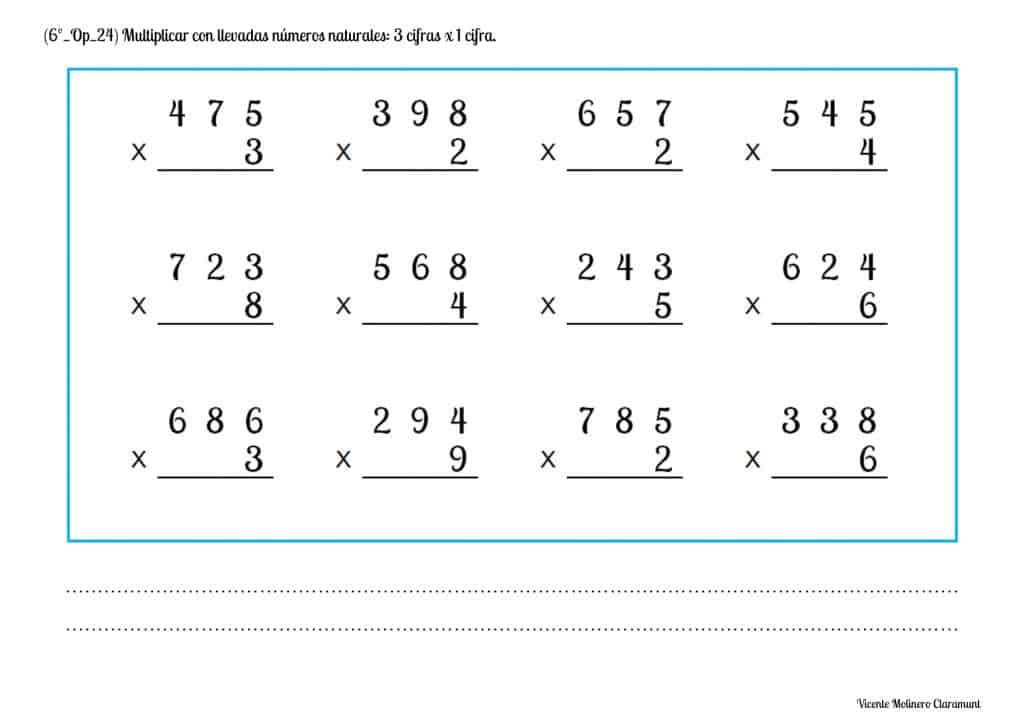#### Solve multiplication word problems with carrying with natural numbers: 3 digits x 1 digit

We present you various multiplication word problems of three figures by one figure (regrouping) so that you can continue developing your calculation skills, your logical-mathematical reasoning and the resolution of problems.

SPANISHSPANISHENGLISH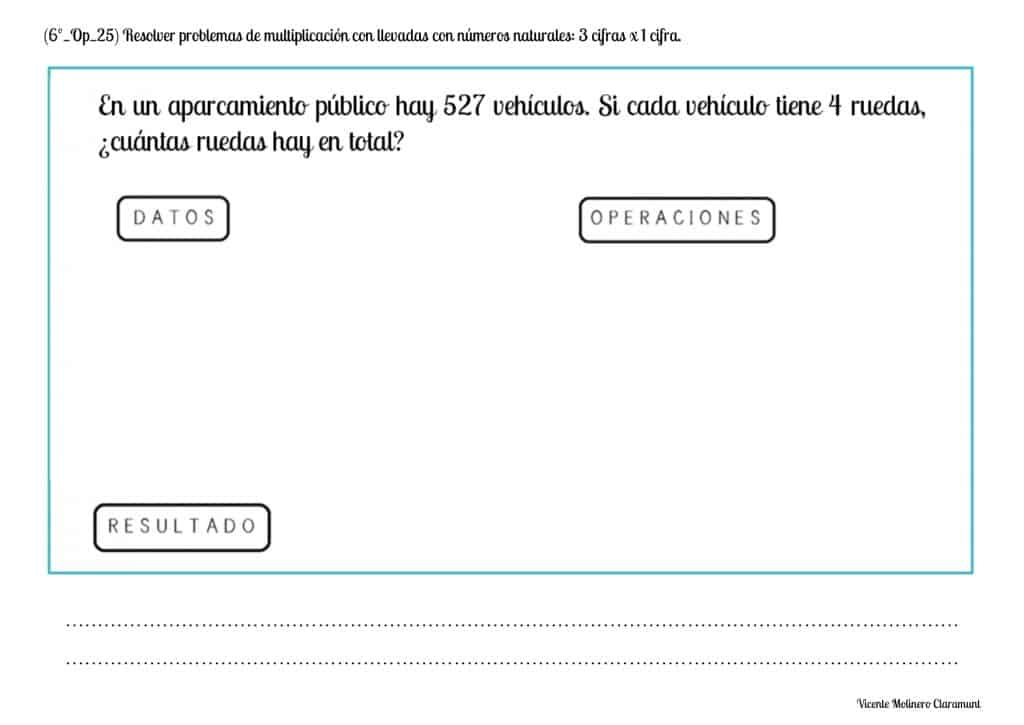#### Multiply natural numbers without carrying: 4 digits by 1 digit

We propose some educational activities with the numbers up to 9,999 in the multiplicand together with their detailed explanation so that you understand step by step how to solve four-digit multiplications for a number without carry.

SPANISHENGLISHSee the detailed explanation of a multiplication without regrouping of 4 digits in the multiplicand and one digit in the multiplier that we propose: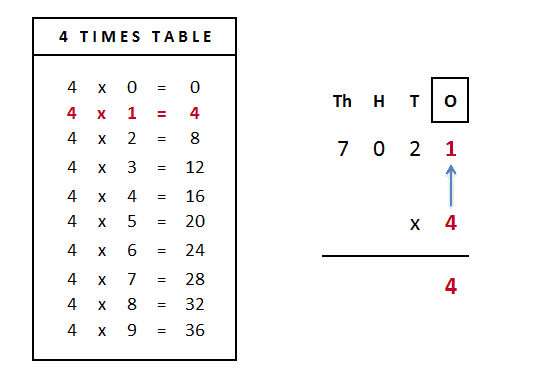Let's multiply 7,021 x 4

1st) We start by multiplying the multiplier (lower digit) by the number of the ones of the multiplicand (upper digit):

4 x 1 = 4 ones

2nd) Next, we will write the result of the multiplication (4) below the horizontal dividing line and aligning it with the figure of the ones.

3rd) We will multiply, on this occasion, the figure of the multiplier (table of 4) by the figure of the tens of the multiplicand:

4 x 2 = 8 tens

4th) Next, we write the result of the multiplication (8) below the figure of the tens in an aligned way and below the horizontal dividing line.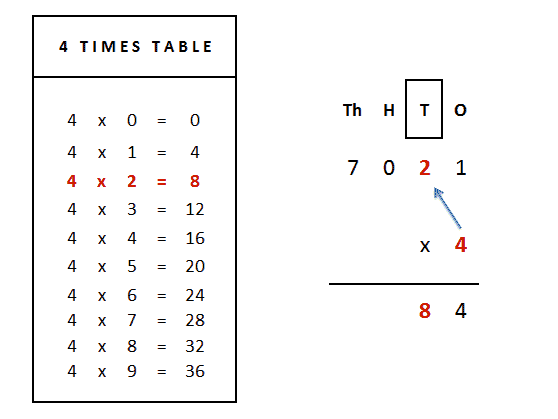5th) We continue multiplying the multiplier (lower figure) by the digit of the hundreds of the multiplicand (upper figure):

4 x 0 = 0 hundreds

6th) Now, we will write the result of the multiplication (0) below the horizontal dividing line and aligning it with the figure of the hundreds.

7th) We will multiply the multiplier (lower figure) by the figure of the thousands of the multiplicand (upper figure):

4 x 7 = 28 thousands

8th) Finally, we write the result of the multiplication (28) below the figure of the thousands and after the horizontal dividing line.

You have finally solved the multiplication! The result: 7,021 x 4 = 28,084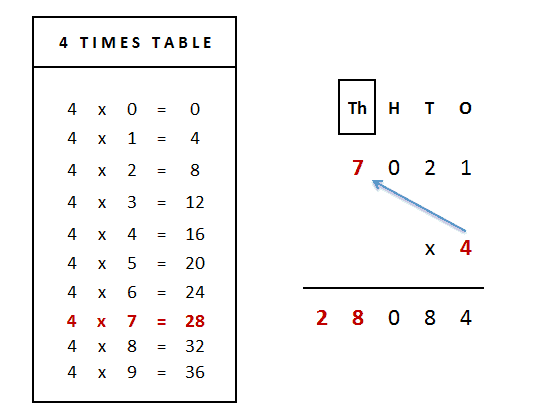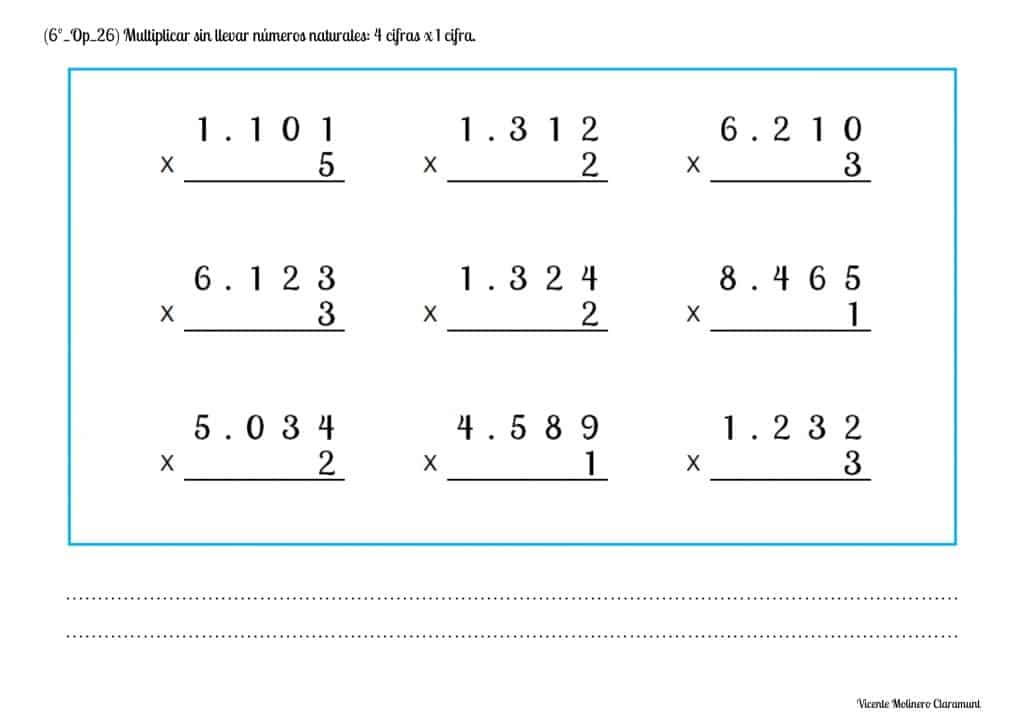#### Solve multiplication word problems without carrying with natural numbers: 4 digits x 1 digit

We provide you with a set of multiplication word problems without carrying of four digits (multiplicand) by one digit (multiplier) so that you can continue to improve your math skills. Previously, we detail what is the process for its adequate resolution through the lessons that we have expressly selected for you.

SPANISHSPANISHENGLISH#### Multiply natural numbers with carrying: 4 digits by 1 digit

We offer you some practical exercises prepared for downloading and printing in PDF with the support of a lesson so that you can easily learn how to solve multiplications carrying of four-digits (multiplicand) by one digit (multiplier).

SPANISHENGLISHWe provide you with an example solved in detail that will help you understand the algorithm for solving multiplication of four figures in multiplicand by one figure in the multiplier:Let's multiply 6,253 x 9

1st) We start by multiplying the multiplier (lower digit) by the number of the ones of the multiplicand (upper digit):

9 x 3 = 27 ones

2nd) The 27 represents 2 tens and 7 ones. We will align the digit 7 below the dividing line (since it corresponds to the ones) and we will save the 2 tens in the tens column to add them in the next step.

3rd) We will multiply, on this occasion, the figure of the multiplier (table of 9) by the figure of the tens of the multiplicand:

9 x 5 = 45 tens

We will add to the result the 2 tens that we had previously saved:

45 + 2 = 47 tens

4th) In the tens column, 47 represents 4 hundreds and 7 tens. We will align the figure 7 below the dividing line (since it corresponds to the tens) and we will save the 4 hundreds in the hundreds column to add them in the next step.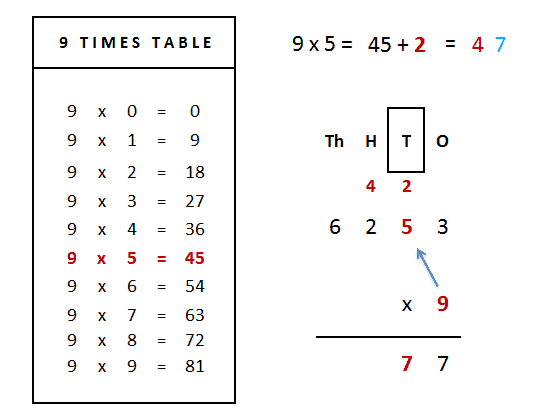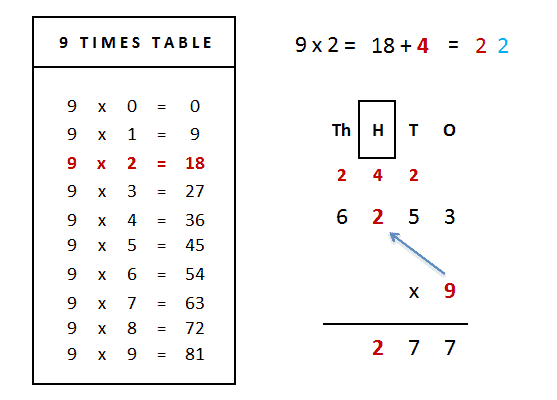5th) We will multiply the digit of the multiplier (table of 9) by the digit of the hundreds of the multiplicand:

9 x 2 = 18 hundreds

We will add to the result the 4 hundreds that we had previously saved:

18 + 4 = 22 hundreds

6th) In the hundreds column, 22 represents 2 hundreds and 2 tens. We will align the figure 2 below the dividing line (since it corresponds to the tens) and we will save the 2 thousands in the thousands column to add them in the next step.

7th) We will multiply the multiplier (lower figure) by the figure of the thousands of the multiplicand (upper figure):

9 x 6 = 54 thousands

We will add to the result the 2 thousands that we had previously saved:

54 + 2 = 56 thousands

4º) Finalmente, escribimos el resultado (56) debajo de la cifra de las unidades de millar y lo alineamos debajo de la línea divisoria horizontal.

You've got it! The result: 6,253 x 9 = 56,277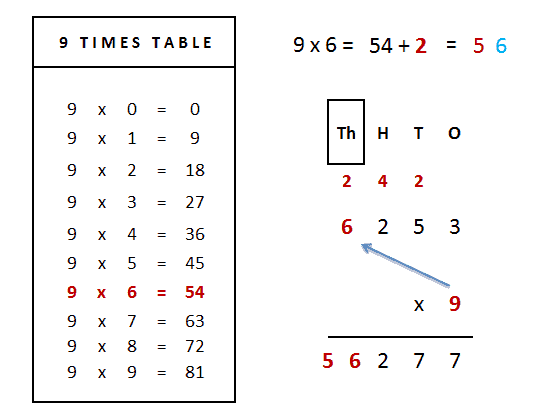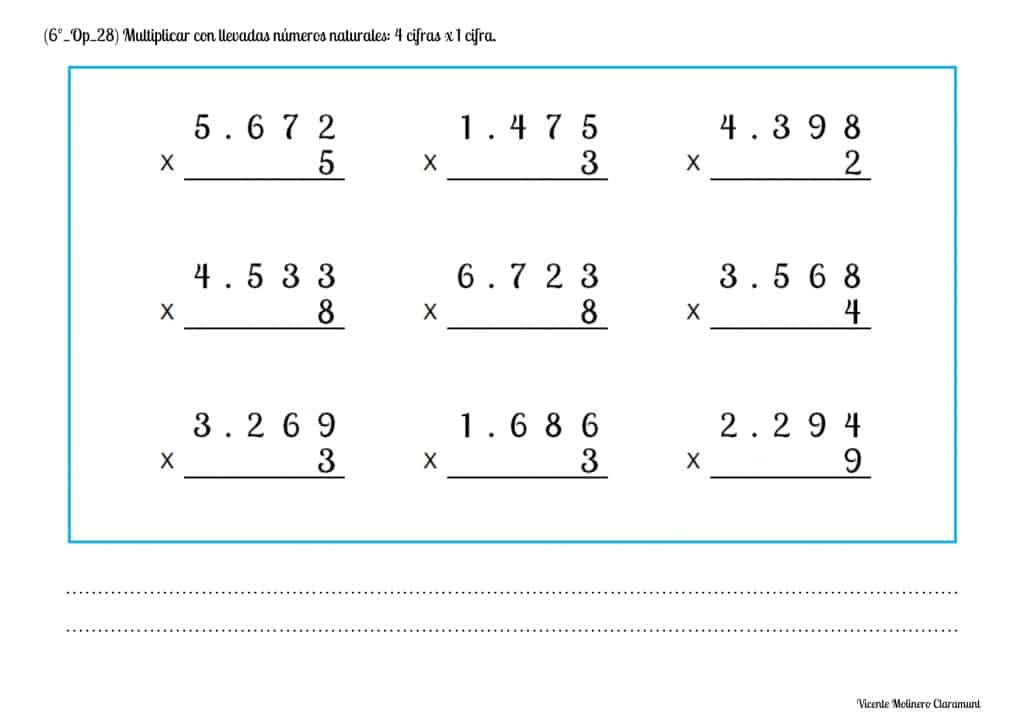#### Solve multiplication word problems with carrying with natural numbers: 4 digits x 1 digit

We offer you pedagogical exercises prepared for downloading and printing in PDF supported by a didactic tutorial so that you can quickly understand how to find the multiplications by carry over of four digits (multiplicand) by one digit (multiplier).

SPANISHENGLISH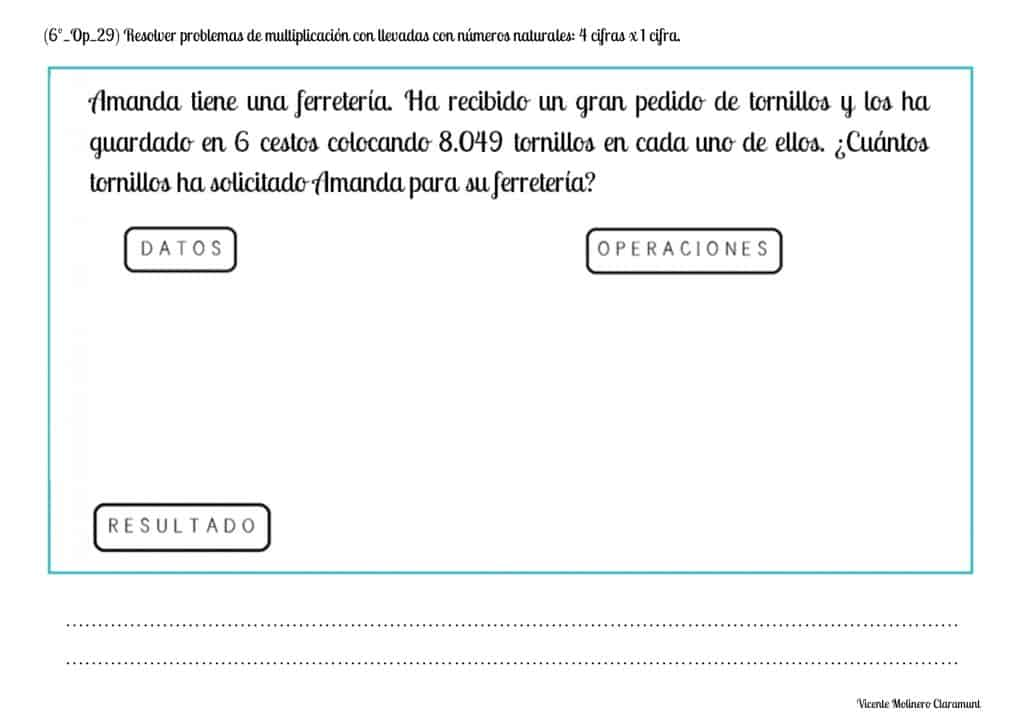#### Multiply natural numbers by 10, 100, 1,000 and so on

Do you know how to multiply a number by the unit followed by zeros? We will explain it to you below!

First, watch the video explanation and learn with simple steps to multiply any natural number by the unit followed by zeros: 10, 100, 1,000, 10,000, 100,000 and so on.

SPANISHENGLISHThe procedure is very simple: only as many zeros are added to the end as there are in the number we have multiplied by, as shown in the examples.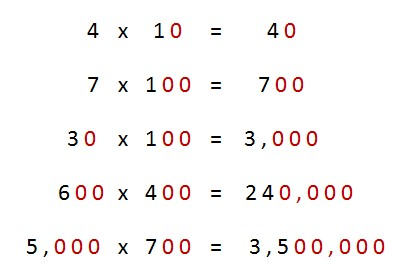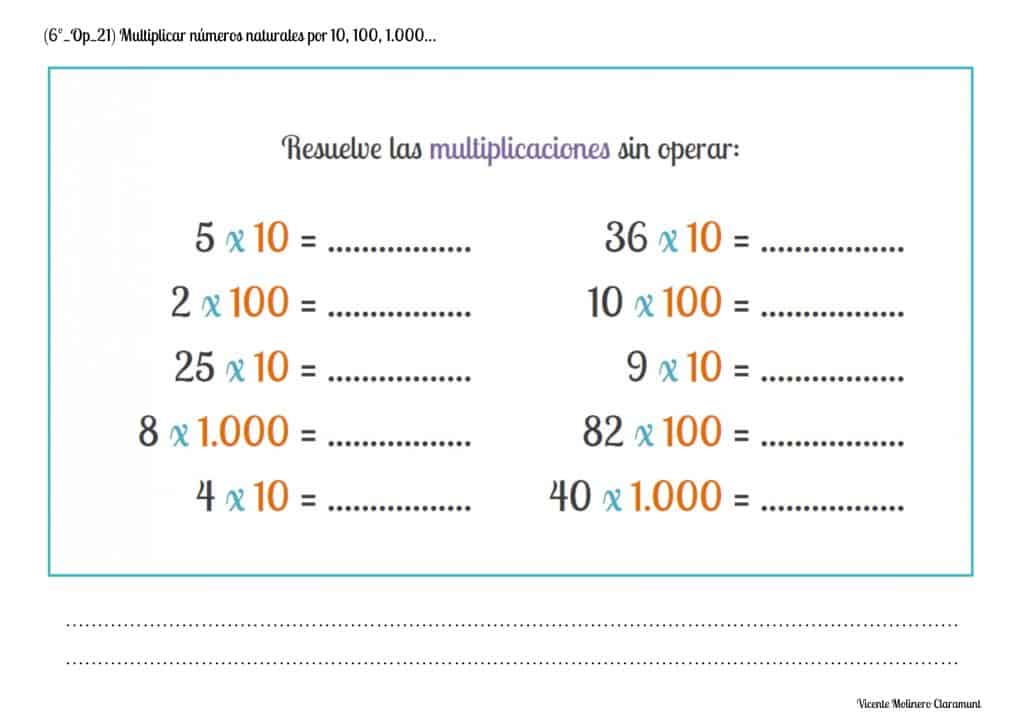#### Mentally calculate simple operations: Multiplication

Here are some mental math activities to test your memory and show that you already know all the multiplication tables.#### Applying the Commutative Property of Multiplication

One of the most important fundamental properties of multiplication is "commutativity":

A x B = B x A

The Commutative Property of Multiplication states that "the order in which the factors are multiplied does not alter the product".

In other words, when we multiply two numbers (factors) with each other, the order in which they are multiplied will not matter because the result (product) will always be the same.

SPANISHENGLISH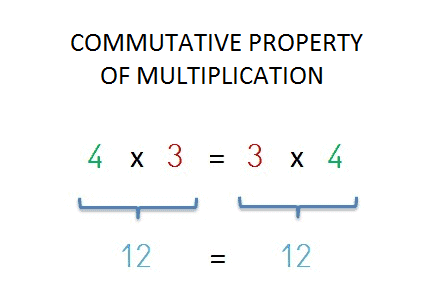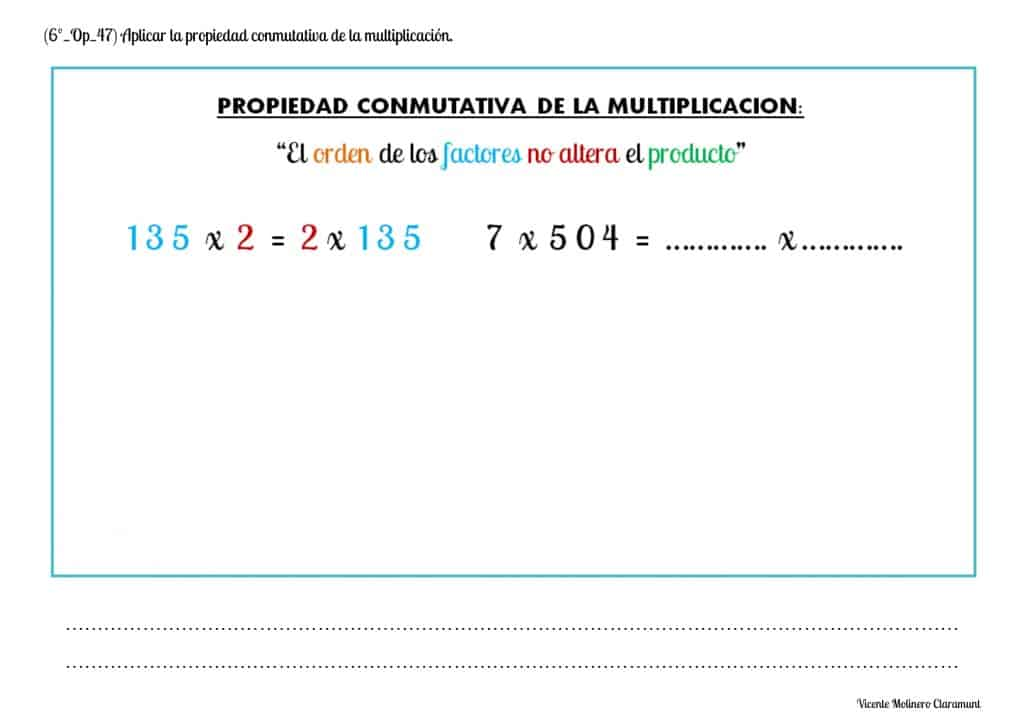#### Apply the Associative Property of Multiplication

Another of the fundamental properties of multiplication is "associativity", where the use of parentheses tells us in what order we must solve the multiplications :

A x ( B x C ) = ( A x B ) x C

The Associative Property of Multiplication determines that "when three or more numbers are multiplied, the result is the same regardless of the order in which the factors are multiplied".

In other words, when we multiply three or more numbers (factors) with each other, the order in which they are multiplied will not matter because the result (product) will always be the same.

SPANISHENGLISH#### Apply the Distributive Property of Multiplication over Addition

We are going to learn yet another one of the fundamental properties of multiplication, in this case, the property called "distributivity of addition", where both the addition and multiplication algorithms are combined through the use of parentheses, which indicate the hierarchy or order in which we must solve the combined operations:

A x ( B + C ) = ( A x B ) + ( A x C )

The Distributive Property of Multiplication over addition states that " the sum of two numbers multiplied by a third number is equal to the sum of each addend multiplied by the third number".

SPANISHENGLISH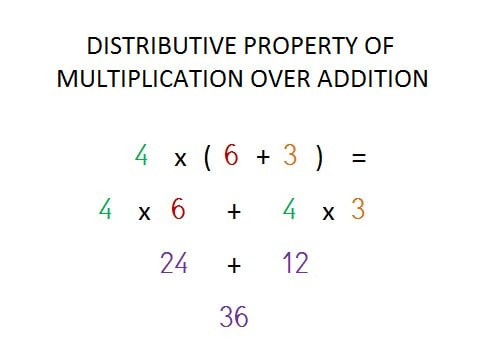#### Apply the Distributive Property of Multiplication over Subtraction

Finally, we will learn the fourth of the fundamental properties of multiplication, the property known as the "distributivity of subtraction", where both the subtraction and multiplication algorithms are combined through the use of parentheses, which indicate the hierarchy or order in which we must solve the combined operations:

A x ( B - C ) = ( A x B ) - ( A x C )

The Distributive Property of Multiplication over subtraction establishes that "the subtraction of two numbers multiplied by a third number is equal to the subtraction of each number multiplied by the third number".

SPANISHENGLISHEvery teacher must become the compass that activates the magnets of curiosity, knowledge and wisdom in his or her students

Ever Garrisson